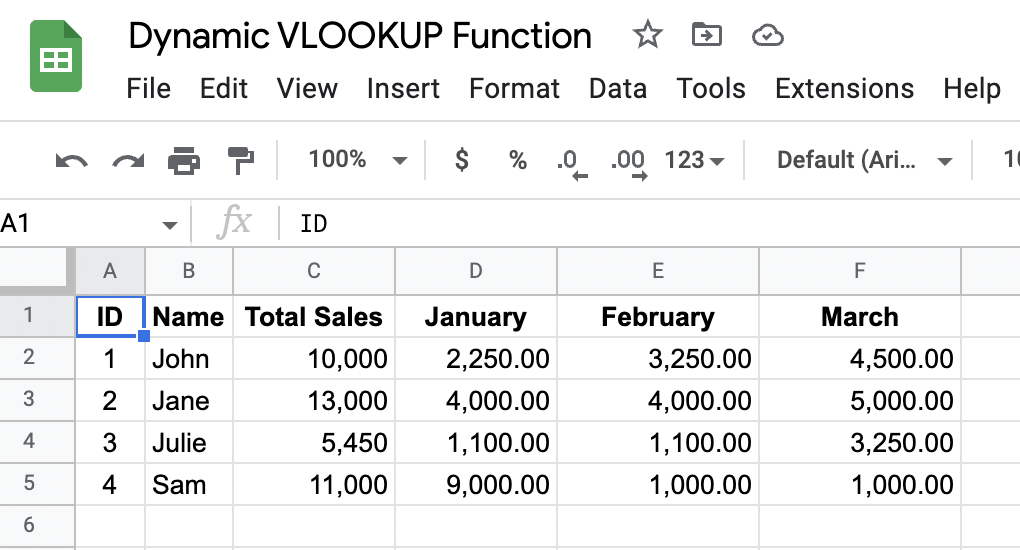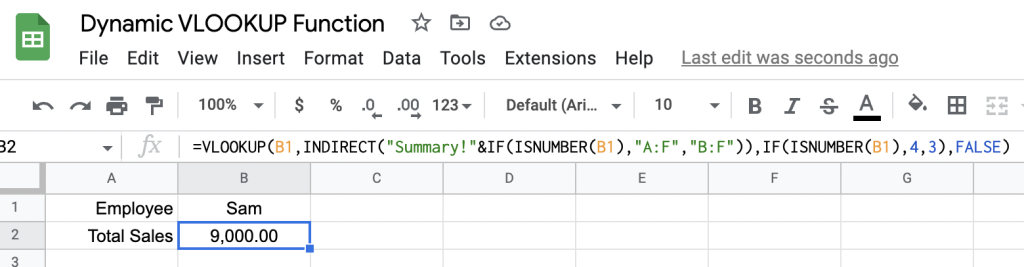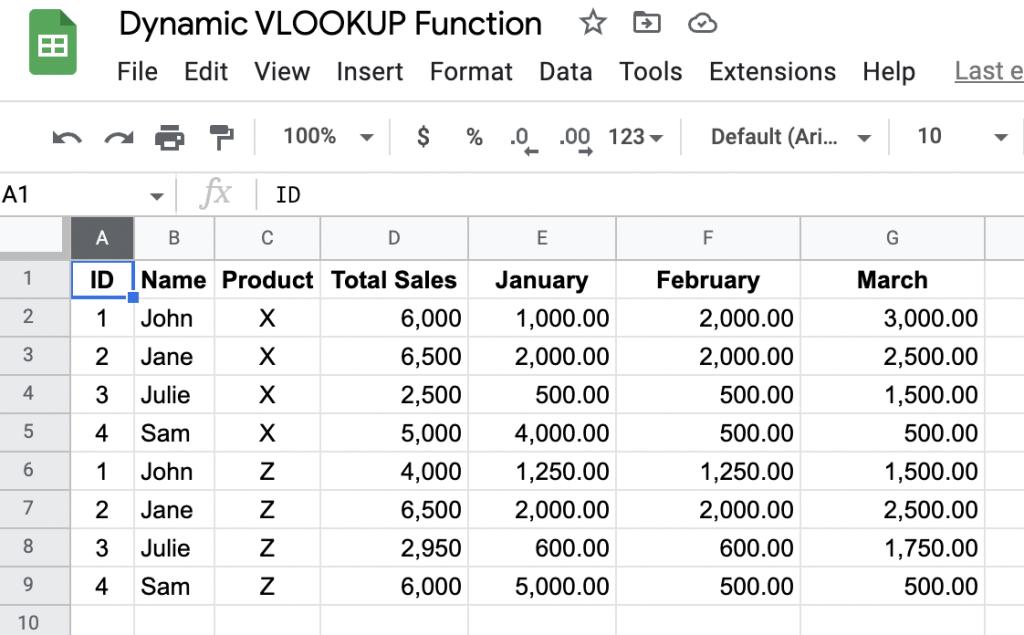# 3 Ways To Make VLOOKUP Dynamic In Google Sheets (Examples)

How do you make the range and column index number in a ``` VLOOKUP ``` function in Google Sheets dynamic?

The ``` VLOOKUP ``` function is a popular formula used in spreadsheets to source data using the first column of a range as the primary key to search the ``` search_key ``` and then to return the intersection of the cell in that row with ``` column_index_number ``` .

Here is the syntax of the ``` VLOOKUP ``` function with its named parameters:

`=VLOOKUP(search_key, range, column_index_number, [is_sorted])`

As stated above the first parameter ``` search_key ``` is the value in the ``` range ``` ‘s first column you want to search, with ``` column_index_number ``` being the column to return from the row matching the ``` search_key ``` . The last optional parameter ``` is_sorted ``` can be set to ``` FALSE ``` to find an exact search for ``` search_key ``` , or ``` TRUE ``` to perform an approximate match of ``` search_key ``` .

(If using ``` TRUE ``` for ``` is_sorted ``` it is best to have your ``` range ``` sorted by the first column in ascending order to prevent getting a wrong return value.)

Knowing the parameters of the ``` VLOOKUP ``` formula can help to determine what aspects you want to make dynamic, and there are three parameters than can dynamically change:

• ``` search_key ``` this can change according to what you would want to search for;
• ``` range ``` this could change according to where you need to search; and
• ``` column_index_number ``` could change according to what value you want to be returned.

Changing the ``` search_key ``` value is trivial, so I’ll explore how to make the ``` VLOOKUP ``` function dynamic by modifying the second and third parameters in this article.

## ``` VLOOKUP ``` Dynamic Ranges

There are multiple approaches to making the ``` range ``` dynamic with the ``` VLOOKUP ``` function. One approach is to use the ``` INDIRECT ``` function, which is useful when the ``` search_key ``` needs to be located in a different column. Another approach is to use the ``` FILTER ``` function, which is useful when the ``` search_key ``` can be found multiple times in the first column of the ``` range ``` .

Here are some examples demonstrating how each approach works.

### Dynamic ``` VLOOKUP ``` With ``` INDIRECT ``` For ``` range ```

As the second parameter in the ``` VLOOKUP ``` function requires a range to make this dynamic, you would need to use the ``` INDIRECT ``` formula .

One reason why you may want to make the ``` range ``` dynamic is to change the field for the primary key according to the type of ``` search_key ``` entered.

For example, if you have a Summary sheet containing the staff IDs, Names, Total Sales YTD and Sales for Each Month, you could make the ``` range ``` dynamic by using ``` INDIRECT ``` function according to the type of search entered for the staff member.

Here’s an example of what the ``` Summary ``` sheet would look like:Summary sheet containing employee ID, name, product, total sales YTD followed by the individual sales of the product each month

To construct a dynamic ``` VLOOKUP ``` range would mean creating a formula like so in a new sheet:

``=VLOOKUP(B1,INDIRECT("Summary!"&IF(ISNUMBER(B1),"A:F","B:F")),IF(ISNUMBER(B1),4,3),FALSE)``

The result is the following when entering the name ``` Sam ``` in cell ``` B1 ``` :VLOOKUP with INDIRECT range to help identify what primary column to use in the range parameter

And here’s the same sheet where the ``` search_key ``` is an employee’s ID:

As you can see with both results, the desired outcome is achieved. However, when making a ``` VLOOKUP ``` formula dynamic when expanding or contracting the ``` range ``` width, you will need to make the ``` column_index_number ``` parameter dynamic too.

When the ``` range ``` of the ``` VLOOKUP ``` formula is modified in height, then the ``` column_index_number ``` can stay the same.

### Dynamic ``` VLOOKUP ``` With ``` FILTER ``` For ``` range ```

Another popular approach is to apply a filter on the ``` range ``` . This is common when the ``` search_key ``` can be found multiple times in the first column of the ``` range ``` .

As the ``` Data ``` sheet contains more than the ``` Summary ``` sheet, for example, including the individual Products sold per month if a result was needed for the total sales made of a specific product the ``` range ``` would need to be compressed to just those rows.

Here’s what the ``` Data ``` sheet looks like:Data sheet contains employee ID, name, product name, total sales YTD, and then individual sales per month

Here’s how the formula would look when using the ``` FILTER ``` function combined with the ``` VLOOKUP ``` :

``=VLOOKUP(B2, FILTER(Data!A:D, Data!C:C=B3), 4, FALSE)``

Here is how the sheet is constructed and it’s result:

As you can see from the above result the ``` FILTER ``` function helps to reduce the rows in ``` range ``` by the conditions placed in the second and subsequent filters. In this case, the ``` Data ``` sheet is reduced by column ``` Data!C:C ``` matching cell ``` B3 ``` .

## ``` VLOOKUP ``` Dynamic Column Index Number

To make the index column in the ``` VLOOKUP ``` function dynamic, use the ``` MATCH ``` formula in the index column parameter. This approach is useful when specific data is needed from a specific column.

With the example spreadsheet used, if you wanted to obtain a specific total amount of sales for a specific month, then you can use the ``` VLOOKUP ``` formula with ``` MATCH ``` to obtain the specific intersection of the found ``` search_key ``` and the matched column index number.

Here’s how this formula would look:

``=VLOOKUP(B2,Summary!A:F,MATCH(B3,Summary!A1:1,0),FALSE)``

Here’s how the result looks in the spreadsheet:

As you can see from the above, the result changes according to the input from the specific month requested. The ``` MATCH ``` formula finds the matching column name in cell ``` B3 ``` and returns the index where this item is found, which is what is needed for the ``` column_index_number ``` .

Instances where it helps to make the ``` column_index_number ``` dynamic will happen when you want to obtain a result from the intersection of the searched row and a specific column.

## Summary

There are 3 approaches you can use to make the ``` VLOOKUP ``` function dynamic. One approach is to modify the ``` range ``` using the ``` INDIRECT ``` formula, which can be helpful when you want to change the field for the primary key according to the type of ``` search_key ``` entered. When using this approach you will need to modify the ``` column_index_number ``` if the returning column needs to stay the same.

Another popular approach is to apply the ``` FILTER ``` function to the ``` range ``` . This is common when the ``` search_key ``` can be found multiple times in the first column of the ``` range ``` , and the data can further be reduced by applying filters.

Finally, another way to make the ``` VLOOKUP ``` function dynamic is by changing just the ``` column_index_number ``` dynamic by using the ``` MATCH ``` formula. This approach is useful when specific data is needed from a specific column.